# C++ Storage Classes

The C++ variables are divided into 4 major storage classes which can change the scope/lifetime of the variables. In this article, you will learn about C++ storage classes with examples.

The C++ storage classes are the same as the C programming language. Here is the list of storage classes.

1. Automatic Storage class
2. Register Storage
3. Static Storage class
4. External Storage class

The storage class controls the scope (local or global) of the variable and lifetime of the variable during program execution. You must use the appropriate keyword for each storage class during variable declaration.

For example, to declare a static storage class variable use the keyword-.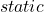.

static int box;

## Automatic Storage Class

The automatic storage class is the default storage class. The C++ program allocates and deallocates automatic storage to all variables. There is no need to declare a variable automatic.

The value of the auto variable is destroyed once its lifetime is over. The same variable gets a new storage when a function or program starts again. Use the keyword auto to declare a variable automatic.

auto int account;

### Example Program: #1

// Demo Auto Storage Class
#include <cstdlib>
#include <iostream>
using namespace std;
int main()
{
// Variable Declaration
auto float a,b,c,d,n;
//initialize n value
n = 0.0;
cout << " Enter A value:";
cin >> a;

cout << "Enter B value:";
cin >> b;

cout << "Enter C value:";
cin >> c;

cout << "Enter D value:";
cin >> d;

//Expression
n = (a * b)/ (c + d);
//Printing Output
cout << "The Value of N =" << " " << n << endl;
system("PAUSE");
return EXIT_SUCCESS;
}

### Output:

Enter A value:2.4
Enter B value:5.3
Enter C value:6.3
Enter D value:1.6
The Value of N = 1.61013

## Register Storage Class

The register storage class make sure that the variable gets stored in a register for faster access. Anything stored in the register is quickly accessed compared to memory. But, this is not guaranteed, it may get stored in memory also.

To declare a register storage class variable, use the following syntax.

### Example Program: #2

//Demo Register Storage Class
#include <cstdlib>
#include <iostream>
#include <cmath>
using namespace std;
int main()
{
//Variable Declaration

//Variable Initialization
Sine_value = 0.0;

cout << "Enter Degrees To Find Sine Value:";
cin >> degree;

//Convert Degree to Radian measure and Computing Sine value

//Printing Output
cout << "Sine Value =" << " " << Sine_value << endl;
system("PAUSE");
return EXIT_SUCCESS;
}

### Output:

Enter Degrees To Find Sine Value:90
Sine Value = 1

## Static Storage Class

The static storage class does not destroy a variable when the function block is terminated. It retains the variable and its value for next time whenever the function is invoked. The new values can replace the old values of static variables. Thus, the lifetime of a variable is increased with the help of keywordThe global variables are static by default, but the local variable inside a block can also be avariable. Here is the syntax to declare a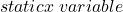.

static int total_value;

### Example Program: #3

This program demonstrates the value of a variable without a static storage class.

//Demo in C++ without static keyword
#include <cstdlib>
#include <iostream>
using namespace std;
int main()
{
//Variable Declaration and function declaration
int bill_1,bill_2;
int i = 0;
int total = 0;
int account_payable(int, int);
while(i < 3)
{
account_payable(bill_1, bill_2) ;
i++;
}

system("PAUSE");
return EXIT_SUCCESS;
}
//Function definition
int account_payable(int bill_1, int bill_2)
{
// Local variable declaration
int sum = 0;
// Variable initialization
bill_1 = 25000;
bill_2 = 35000;
sum = sum + bill_1 + bill_2;
cout << "Sum = " << " " << sum << endl;
}

### Output:

Sum =  60000
Sum =  60000
Sum =  60000

The output is 60000 because each iteration of the loop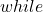in the program, the variable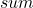is destroyed. It then starts the next iteration with a new variable.

Let us examine the output of the same program withkeyword.

### Example Program: #4

//Demo Static Variable in C++ with static keyword
#include <cstdlib>
#include <iostream>
using namespace std;
int main()
{
//Variable Declaration and function declaration
int bill_1,bill_2;
int i = 0;
int total = 0;
int account_payable(int, int);
while(i < 3)
{
account_payable(bill_1, bill_2) ;
i++;
}
system("PAUSE");
return EXIT_SUCCESS;
}
//Function definition
int account_payable(int bill_1, int bill_2)
{
// Local variable declaration
static int sum = 0;
// Variable initialization
bill_1 = 25000;
bill_2 = 35000;
sum = sum + bill_1 + bill_2;
cout << "Sum = " << " " << sum << endl;
}

### Output:

Sum =  60000
Sum =  120000
Sum =  180000

With static keyword, the variable sum is not destroyed and the new value of each iteration is added to the previous value. Hence, there is an incremental increase in the output.

## External Storage Class

The variables declared in the global declaration section which is located above the main function. Such a variable is an external variable. But, there are two types of external variable. An external variable without keyword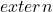. The scope is limited to current C file.

The external storage class allow variables to share information across files. The variable is declared in some file called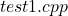. But, it is used in another file called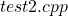.

To declare a variable use thekeyword.

### Example Program: #5

/*
Demo For External Storage Class
File Name: test1.cpp
*/
#include <cstdlib>
#include <iostream>
//Gloabl declaration of extern int amount before usage
int amount = 13000;
using namespace std;
int main()
{
//Variable Declaration
int expenses, total;
//Initialization
expenses = 1000;
total = 0;

//Calculate total amount
total = expenses + amount;
cout << "Total Amount =" << " " << total << endl;
system("PAUSE");
return EXIT_SUCCESS;
}

The code for second file –.

/* Demo external storage class
File Name: test2.cpp
*/
#include <iostream>
extern int amount;

### Output:

Total Amount = 14000